# Witt algebra

Jump to: navigation, search

Letbe a field of characteristic. Consider the-algebra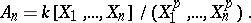Let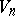be the algebra of-derivations of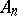. The algebra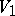is known as the Witt algebra. The() are known as the split Jacobson–Witt algebras. The algebrais a simple Lie algebra, except when it is-dimensional. The dimension ofis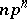.

More generally one considers the-algebras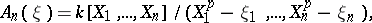and their algebras of derivations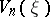, the Jacobson–Witt algebras. Theandare (obviously)-forms ofand, where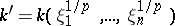(cf. Form of an (algebraic) structure). Many simple Lie algebras in characteristicarise as subalgebras of the.

Letbe an additive group of functions on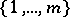intosuch that the only elementofsuch that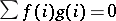for all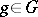is the zero element. For instance,can be the set of all functions fromto some additive subgroup of. Ifis finite, it is of order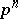for some. Now, letbe a vector space overwith basis elements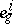,,, and define a bilinear product onby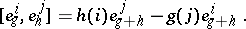There results a Lie algebra, called a generalized Witt algebra. Ifis finite of order, the dimension ofis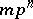, andis a simple Lie algebra if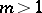or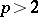.

Ifis of characteristic zero,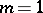andis the additive subgroup, the same construction results in the Virasoro algebra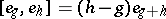.

Ifis of characteristicandis the group of all functions onwith values in, one recovers the Jacobson–Witt algebras.

There are no isomorphisms between the Jacobson–Witt algebrasand the classical Lie algebras in positive characteristic when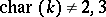. Several more classes of simple Lie algebras different from the classical ones and theare known, [a1].

The Witt algebra(s) described here should of course not be confused with the Witt ring of quadratic forms over a field, nor with the various rings of Witt vectors, cf. Witt vector.